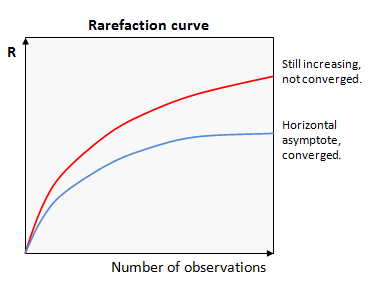Rarefaction

Rarefaction is a technique used in numerical ecology. Most commonly, rarefaction is used to determine whether all the species in an ecosystem have been observed, as discussed in this Wikipedia article.

More generally, the goal of rarefaction is determine whether sufficient observations have been made to get a reasonable estimate of a quantity (call it R) that has been measured by sampling. The most commonly considered quantities are species richness (the number of different species in an environment or ecosystem) and alpha diversity (a measure of species diversity that may attempt to extrapolate to larger numbers of samples and/or take into account the abundance distribution -- there are many different definitions).

The basic idea of rarefaction is to plot the value of the measured quantity R against the number of observations used in the calculation. Values of R for smaller numbers of observations are obtained by taking random subsets. If we get a similar value of R with fewer observations, then it is reasonable to infer that R has converged on a good estimate of the correct value. Conversely, if R is systematically increasing or decreasing as more samples are added, then we can infer that we cannot make a good estimate of R. These two cases are shown in the figure below. In this example, the upper curve (red) is still increasing, so has not converged. The lower curve (blue) has reached a horizontal asymptote, so we can infer that the value of R is a good estimate of the value that would be obtained with the maximum possible number of observations (e.g., every individual in the ecosystem observed once).

If R does not converge, there are two possibilities: we need more samples to get a good estimate, e.g. because we have not yet sampled all the taxa present, or undetected read errors are systematically biased towards increasing R, in which case the measured R might increase indefinitely. This effect is commonly seen with the number of OTUs. Suppose there is a fixed probability that a read has >3% bad bases and will thus induce a spurious OTU. As the number of reads increases, the number of OTUs will increase due to these bad reads, regardless of whether all the species in the sample have been detected. The number of OTUs will therefore never converge.

This type of plot is called a "rarefaction curve". Note that the conclusions we can draw from a rarefaction curve are suggestive but not definitive -- there could be rare species that have not yet been observed even if the curve appears to converge.

There is a standard formula for calculating the abundance rarefaction curve of species (or OTUs) given the observed abundance of each species (or number of reads for each OTU), but this does not apply if singleton reads are discarded, as recommended in the UPARSE pipeline. See abundance rarefaction for discussion.